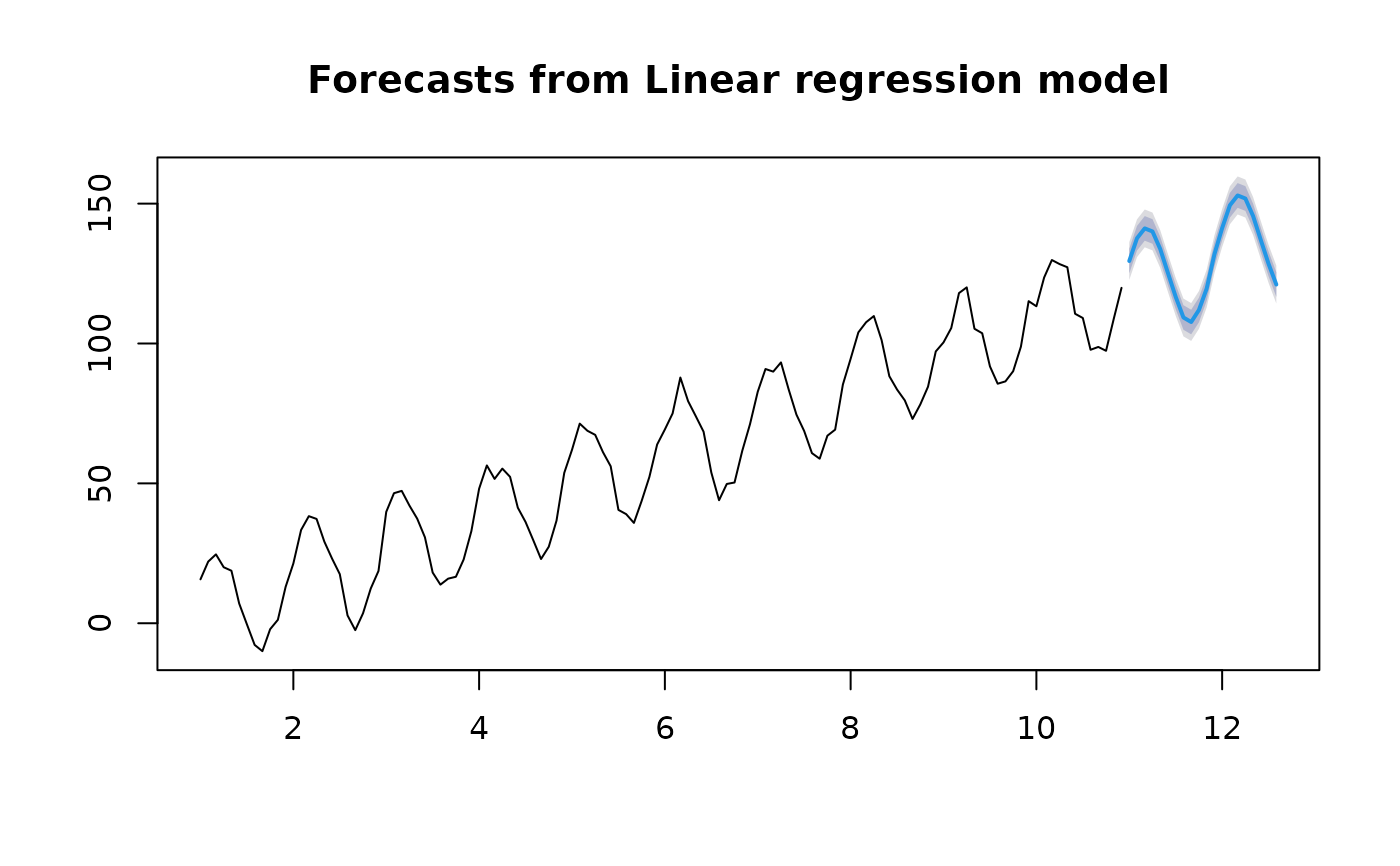forecast.lm is used to predict linear models, especially those involving trend and seasonality components.

# S3 method for lm
forecast(
object,
newdata,
h = 10,
level = c(80, 95),
fan = FALSE,
lambda = object\$lambda,
ts = TRUE,
...
)

## Arguments

object Object of class "lm", usually the result of a call to lm or tslm. An optional data frame in which to look for variables with which to predict. If omitted, it is assumed that the only variables are trend and season, and h forecasts are produced. Number of periods for forecasting. Ignored if newdata present. Confidence level for prediction intervals. If TRUE, level is set to seq(51,99,by=3). This is suitable for fan plots. Box-Cox transformation parameter. If lambda="auto", then a transformation is automatically selected using BoxCox.lambda. The transformation is ignored if NULL. Otherwise, data transformed before model is estimated. Use adjusted back-transformed mean for Box-Cox transformations. If transformed data is used to produce forecasts and fitted values, a regular back transformation will result in median forecasts. If biasadj is TRUE, an adjustment will be made to produce mean forecasts and fitted values. If TRUE, the forecasts will be treated as time series provided the original data is a time series; the newdata will be interpreted as related to the subsequent time periods. If FALSE, any time series attributes of the original data will be ignored. Other arguments passed to predict.lm().

## Value

An object of class "forecast".

The function summary is used to obtain and print a summary of the results, while the function plot produces a plot of the forecasts and prediction intervals.

The generic accessor functions fitted.values and residuals extract useful features of the value returned by forecast.lm.

An object of class "forecast" is a list containing at least the following elements:

model

A list containing information about the fitted model

method

The name of the forecasting method as a character string

mean

Point forecasts as a time series

lower

Lower limits for prediction intervals

upper

Upper limits for prediction intervals

level

The confidence values associated with the prediction intervals

x

The historical data for the response variable.

residuals

Residuals from the fitted model. That is x minus fitted values.

fitted

Fitted values

## Details

forecast.lm is largely a wrapper for predict.lm() except that it allows variables "trend" and "season" which are created on the fly from the time series characteristics of the data. Also, the output is reformatted into a forecast object.

tslm, lm.

Rob J Hyndman

## Examples


y <- ts(rnorm(120,0,3) + 1:120 + 20*sin(2*pi*(1:120)/12), frequency=12)
fit <- tslm(y ~ trend + season)
plot(forecast(fit, h=20))